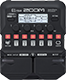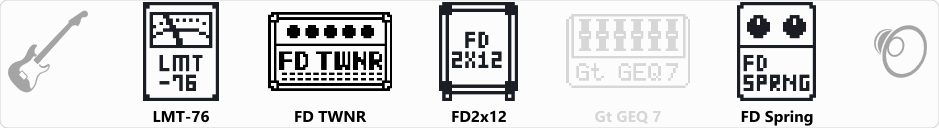# TwinReverb LD

Discussion in 'Zoom G1/G1X Four' started by Mississippi, Apr 1, 2022.

1. TwinReverb LDDevice: Zoom G1 Four
Firmware: 2.00

Name on device: TwinReverb
Optimized for: Phones/Speaker

Effects chain:Bluesy clean tone for humbucker equipped guitars

Effect: "LMT-76" (Dynamics), active - "yes"
"Input" = 41
"Ratio" = 4:1
"REL" = 20
"Output" = 35

Effect: "FD TWNR" (Amp simulator), active - "yes"
"Bass" = 70
"Middle" = 65
"Treble" = 54
"Bright" = ON
"Gain" = 38
"Volume" = 100
"Depth" = 10
"Speed" = 46

Effect: "FD2x12" (Cabinet), active - "yes"
"MIC" = ON
"D57: D421" = 50
"Hi" = 60
"Lo" = 53

Effect: "Gt GEQ 7" (Filter), active - "no"
"100" = 0.0
"200" = 0.0
"400" = 0.0
"800" = 2.0
"1.6k" = 2.5
"3.2k" = 2.0
"6.4k" = 0.0
"VOL" = 80

Effect: "FD Spring" (Reverb), active - "yes"
"Color" = 1
"Lo" = 65
"Hi" = 42
"Mix" = 9

Patch Volume: 120